# Chain Reaction, Nuclear

The following article is from The Great Soviet Encyclopedia (1979). It might be outdated or ideologically biased.

## Chain Reaction, Nuclear

a nuclear reaction in which the particles that cause the reactions are formed as products of the reactions. Thus far, the only known nuclear chain reaction is the fission of uranium and some transuranium elements, such as 239Pu, under the action of neutrons. After the discovery of the fission of nuclei by neutrons by the German scientists O. Hahn and F. Strassmann in 1939, it was demonstrated by J. F. Joliot-Curie and co-workers, by E. Fermi, W. Zinn, and L. Szilard (United States), and by G. N. Flerov that more than one neutron is emitted upon fission of a nucleus:

(1) n + U → A + B + nv

Here, A and B are fission fragments with mass numbers of A ranging from 90 to 150 and v > 1 is the number of fission neutrons. A nuclear chain reaction was first achieved by Fermi in 1942.

Let us assume that only a part f of the total number of fission neutrons can be used to continue the fission reaction. Then, for every neutron of the first generation that induces fission, there will be K = vf neutrons of the next generation that will induce fission, and if K, which is called the neutron multiplication factor, is greater than unity, the number of such neutrons will increase with time t according to the law n = n0e(K –1)t, where τ is the lifetime of the generation of neutrons. If K = 1, the number of fissions per unit time is constant, and a self-sustaining nuclear chain reaction can be achieved. A device in which a controlled self-sustaining nuclear chain reaction occurs is called a nuclear reactor. At sufficiently large values of K – 1, the reaction ceases to be controllable and may lead to a nuclear explosion.

Let us consider nuclear chain reactions based on natural uranium, which for all intents and purposes has two isotopes: 238U (99.29 percent) and 235U (0.71 percent); the amount of 234U is negligible. A 238U nucleus is fissioned only by fast neutrons, with energy ℰ > 1 megaelectron volts (MeV) and a small cross section σf = 0.3 barn. On the other hand, a 235U nucleus is fissioned by neutrons of any energy, the cross section for fission σf(5) increasing sharply with decreasing ℰ. Upon the fission of 238U or 235U by a fast neutron, v ~ 2.5 neutrons are emitted with energy ranging from 0.1 to 14 MeV. This means that a nuclear chain reaction could develop in natural uranium if there were no losses. However, there are losses: a nucleus of 238U may capture neutrons with the formation of 239U (seeRADIATIVE CAPTURE OF ELECTRONS). Furthermore, upon collision of neutrons with a nucleus of 238U, inelastic scattering occurs, in which the energy of the neutrons drops below 1 MeV and thus they can no longer produce fission in 238U. Most of these neutrons undergo radiative capture or escape. Because of this, a nuclear chain reaction cannot develop under these conditions. To produce a nuclear chain reaction in natural uranium, neutrons are slowed down by collisions with light nuclei, such as 2H, l2C, and other moderators (seeNEUTRONS, MODERATION OF). It has been found that the cross section for fission in 235U by thermal neutrons is σf(5) = 582 barns; the radiative capture cross section in 235U (with formation of 236U) is σr(5) = 100 barns, and in 238U, σr(8) = 2.73 barns. Upon fission by thermal neutrons, v = 2.44. This implies that the number of neutrons η that can produce fission in 235U for each absorbed thermal neutron of the preceding generation is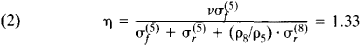Here, ρ85 is the ratio of concentrations of 238U and 235U. This means that a nuclear chain reaction can develop in a mixture of natural uranium with a moderator.

However, in the case of fission by thermal neutrons, fast neutrons are produced that may be absorbed before slowing to thermal energy. The radiative capture cross section for 238U is resonant in nature; that is, it reaches very high values in certain narrow energy bands. The part played by resonance absorption in a nuclear chain reaction by thermal neutrons in homogeneous mixtures of uranium and moderators was first studied by Ia. B. Zel’dovich and Iu. B. Khariton in 1940. In a homogeneous mixture, the probability of resonance absorption is too high for a nuclear chain reaction by thermal neutrons to occur. This difficulty is overcome by distributing the uranium in the moderator in separate blocks stacked in the form of a cube. Resonance absorption of neutrons in such a heterogeneous system decreases sharply for two reasons: (1) the cross section of resonance absorption is so great that the neutrons incident on a block are absorbed in the surface layer, and therefore the inside of the block is shielded and a considerable part of the uranium atoms does not participate in resonance absorption; (2) neutrons of resonance energy that are formed in the moderator may not reach the uranium and may “leave” the dangerous energy interval as they slow down upon scattering by the moderator nuclei. Upon absorption of a thermal neutron in a block, η fast fission neutrons are produced, each of which will cause a small number of fissions of 238U before leaving the block. As a result, the number of fast neutrons emitted from the block into the moderator is equal to єη, where є is the fast fission factor. φ is the resonance escape probability, then only εηφ neutrons are slowed to thermal energy. Some of the thermal neutrons are absorbed in the moderator. Let θ be the probability that a thermal neutron will be absorbed in uranium (thermal utilization). In a homogeneous system,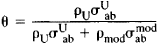In a heterogeneous system,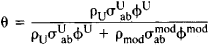Here, ρU and ρmod are the concentrations of uranium and moderator, the σab are the corresponding absorption cross sections, and the Φ are the neutron fluxes. As a result, for every thermal neutron of the first generation that produces fission, we get Kef = ∊ηφθ neutrons of the next generation that can produce fission. Let K be the neutron multiplication factor in an infinite heterogeneous system. If K – 1 > 0, the fission reaction in an infinite crystal lattice will rise exponentially.

If the system has finite dimensions, some of the neutrons may leave the medium. Let us denote the fraction of neutrons that escape by 1 – P; then, Kef = K ~ P neutrons remain to continue the fission reaction, and if Kef > 1, then the number of fissions increases exponentially and the reaction is self-sustaining. Since the number of fissions and consequently the number of fission neutrons in the multiplying medium is proportional to its volume and emission (leakage) of these neutrons is proportional to the surface of the surrounding medium, a nuclear chain reaction is possible only in a medium of rather large dimensions. For example, for a sphere of radius R, the ratio of volume to surface is equal to R/3, and consequently the greater the radius of the sphere, the less will be the neutron leakage. If the radius of the multiplying medium becomes great enough so that a steady-state chain reaction occurs in the system, that is, Kef – 1 = 0, such a system is said to be critical, and its radius is called the critical radius.

To achieve nuclear chain reactions in natural uranium by thermal neutrons, substances with a low radiative capture cross section, such as graphite or heavy water (D20), are used. Nuclear chain reactions in natural uranium are impossible with an ordinary water moderator because of the high absorption of neutrons in hydrogen.

The lifetime of one generation of neutrons must be sufficiently long enough to make possible control of the intensity of a nuclear chain reaction. The lifetime τ0 of thermal neutrons is short, 10–3 sec. However, in addition to prompt neutrons (neutrons emitted from the nucleus within 10–16 sec after fission), there is also a small fraction μ of delayed neutrons that are emitted after the beta decay of fission fragments, with an average lifetime τdel = 14.4 sec. For delayed neutrons accompanying fission in 235U, µ ≈ 0.75 × 10–2. If Kef > 1 + µ, the e-folding time T of the nuclear chain reaction (equal to the time in which the number of neutrons increases by a factor of e) is determined by the relation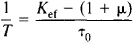that is, delayed neutrons do not take part in the development of a nuclear chain reaction. Of practical importance is the other limiting case: Kef – 1 ≪ µ; then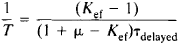that is, prompt neutrons do not play a part in the development of the reaction. Thus, if Kef < 1 + µ, then a nuclear chain reaction will develop with the participation of delayed neutrons over a time of the order of minutes and will be easily controllable. The role of delayed neutrons was first noted by Zel’dovich and Khariton (1940).

A nuclear chain reaction can also be achieved using uranium enriched with 235U, as well as pure 235U. In these cases, it is generated by fast neutrons as well. Upon absorption of neutrons in 238U, 239Np is formed, from which 239Pu is produced after two beta decays. The 239Pu splits up under the action of thermal neutrons, with v = 2.9. Neutron irradiation of 232Th produces 233U that is fissionable by thermal neutrons. In addition, a nuclear chain reaction is possible in 241Pu and odd-numbered isotopes of Cm and Cf. Of the v neutrons formed in one fission event, one is expended on continuation of the nuclear chain reaction, and if losses are reduced, more than one neutron may be retained for nuclear fuel conversion, which may lead to breeding of fuel.

### REFERENCES

Galanin, A. D. Teoriia iadernykh reaktorov na teplovykh neitronakh, 2nd ed. Moscow, 1959.
Weinberg, A., and Wigner, E. Fizicheskaia teoriia iadernykh reaktorov. Moscow, 1961. (Translated from English.)
Zel’dovich, Ia. B., and Khariton, Iu. B. Zhurnal eksperimental’noi i teoreticheskoi fiziki, 1940, vol. 10, issue 1, pp. 29–36; issue 5, pp. 477–82.
Fermi, E. Nauchnye trudy, vol. 2. Moscow, 1972. Page 308.

P. E. NEMIROVSKII

The Great Soviet Encyclopedia, 3rd Edition (1970-1979). © 2010 The Gale Group, Inc. All rights reserved.
Site: Follow: Share:
Open / Close